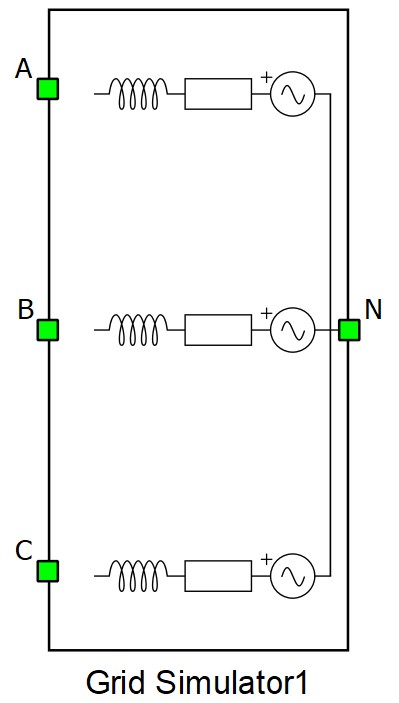# Grid simulator

Description of the Grid Simulator component in Schematic Editor

The Grid Simulator is a component which consists of three single phase voltage sources, series resistances and inductances. It is used to simulate a three phase symmetric or asymmetric grid.

The internal structure of the Grid Simulator is shown in Figure 1. Phase voltage, line voltage and phase current measurements are included, as shown in Figure 1. In Table 1 the schematic symbol and properties window of grid simulator are shown.

Table 1. Grid Simulator in HIL Toolbox
component component dialog window component parametersGrid Simulator• Model definition: R-L, S-U, or S-U and X/R
• Nominal line voltage (V)
• Nominal frequency (Hz)
• Resistance per phase (Ω)
• Inductance per phase (H)
• Short circuit capacity (VA)
• Inductive power factor
• X/R ratio

The list of measurements available in the grid simulator is shown in Internal analog measurements of the grid simulator.

## Calculation from S-U to R-L

$R=\frac{pf\bullet {{V}_{b}}^{2}}{{S}_{b}}$

$L=\frac{\frac{{{V}_{b}}^{2}}{{S}_{b}}\bullet {\left(1-{pf}^{2}\right)}^{0.5}}{2\bullet \pi \bullet f}$

## Calculation from S-U and X/R to R-L

$R=\frac{{{V}_{b}}^{2}}{{S}_{b}\bullet {\left(1+{\left(X/R\right)}^{2}\right)}^{0.5}}$

$L=R\bullet \frac{X/R}{2\bullet \pi \bullet f}$

• R - resistance
• L - inductance
• Vb - nominal line voltage
• f - frequency
• Sb - short circuit capacity
• pf - inductive power factor
• X/R - x/r ratio

## Internal analog measurements of the grid simulator

Analog output variable name Description
(name).I_phase_A Phase A current.
(name).I_phase_B Phase B current.
(name).I_phase_C Phase C current.
(name).V_phase_A Phase A voltage.
(name).V_phase_B Phase B voltage.
(name).V_phase_C Phase C voltage.
(name).V_source_phase_A Phase A source voltage.
(name).V_source_phase_B Phase B source voltage.
(name).V_source_phase_C Phase C source voltage.
(name).V_AB A-B line voltage.
(name).V_BC B-C line voltage.
(name).V_CA C-A line voltage.